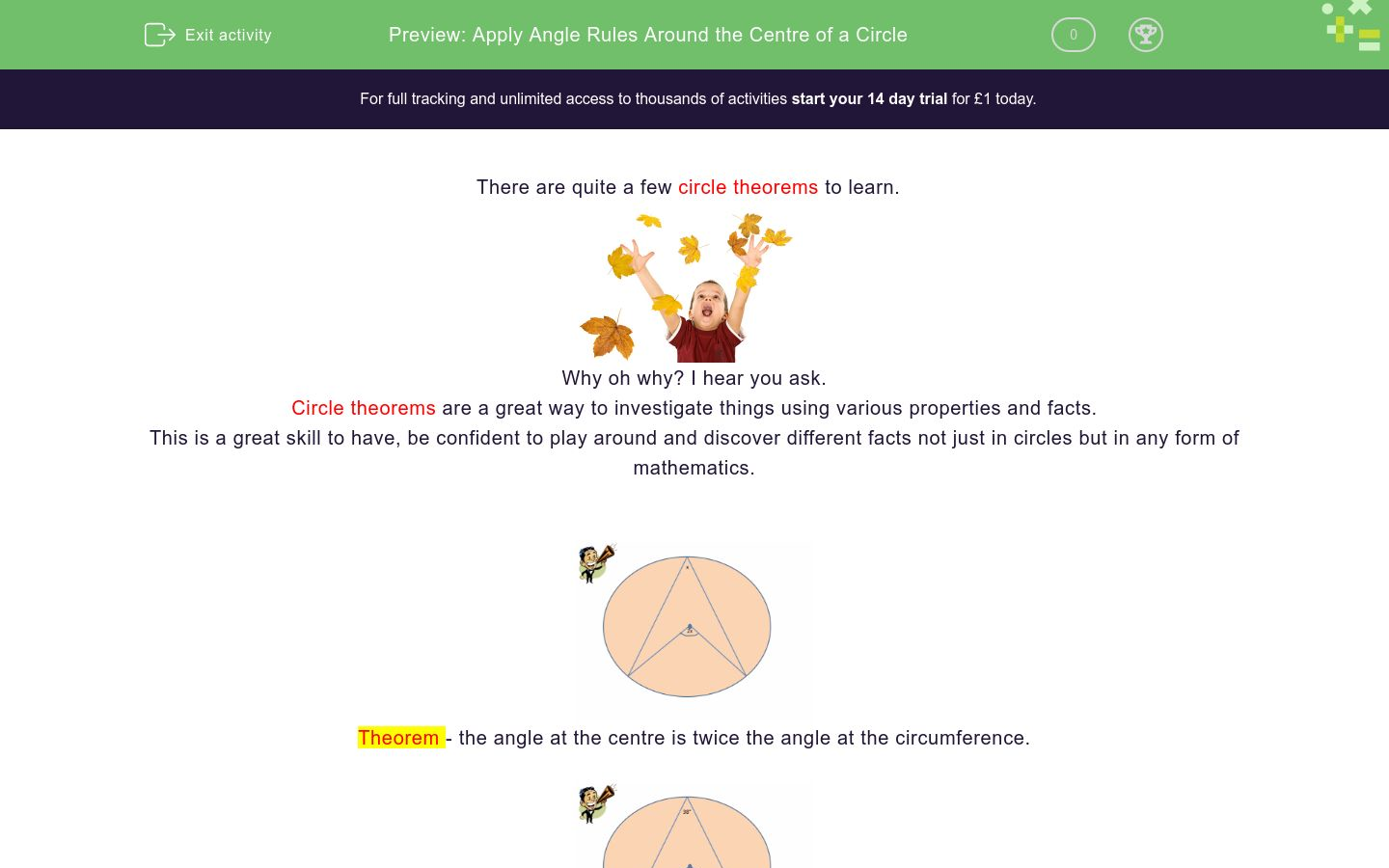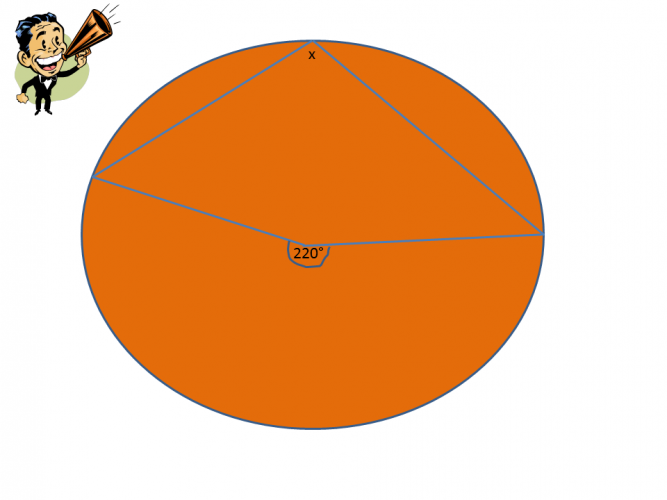# Apply Angle Rules Around the Centre of a Circle

In this worksheet, students will learn about angles at the centre and the circumference and put the rules into practice.Key stage:  KS 4

GCSE Subjects:   Maths

GCSE Boards:   OCR, AQA, Eduqas, Pearson Edexcel

Curriculum topic:   Basic Geometry, Geometry and Measures

Curriculum subtopic:   Circles, Properties and Constructions

Difficulty level:### QUESTION 1 of 10

There are quite a few circle theorems to learn.Why oh why? I hear you ask.

Circle theorems are a great way to investigate things using various properties and facts.

This is a great skill to have, be confident to play around and discover different facts not just in circles but in any form of mathematics.Theorem - the angle at the centre is twice the angle at the circumference.Example - The angle at the circumference is 38°. Applying this theorem 38 x 2 = 76°

So what are you waiting for?Sometimes you have to recall other angle properties.

This is all part of the investigation of circles. Look out for these.What is the value of x?

92°

138°

88°

46°Calculate angle x.35° 105° 145° 70° Angle x is110° 140° 70° 80° Angle x isWhat is the value of x?

30°

60°

90°

110°30°

60°

90°

110°Find the value of x.What are the two correct answers

y = 2x

y = 1/2x

x = 1/2y

x = 2yFind the value of x.

• Question 1What is the value of x?

92°
EDDIE SAYS
The rule is the angle at the centre is twice that on the circumference. Therefore 46 x 2 = 92°
• Question 2Calculate angle x.

44
EDDIE SAYS
Oh, here you have the been given the angle at the centre. So work this the other way. If the angle at the centre is twice that at the circumference the the angle at the circumference is half that at the centre. 88 ÷ 2 = 44°
• Question 3EDDIE SAYS
The angle at the centre is twice that of the circumference therefore 28 x 2 = 56° By the way who invented the round table? Sir cumference.
• Question 435° 105° 145° 70° Angle x is
EDDIE SAYS
Now this is a bit sneaky as this has been presented in a slightly different way. It looks a little bit like the bow tie in the angles in the same segment theorem. x is at the centre and the other angle is on the circumference. 35 x 2 = 70°
• Question 5110° 140° 70° 80° Angle x is
EDDIE SAYS
Another sneaky way of presenting this theorem as it could be mistaken for angles in a cyclic quadrilateral. Start at the centre and look at what other clues you have been given. The angle at the circumference is half that at the centre so therefore 110° Thinking of clues, what question does Sherlock Holmes ask when he is bored? Watson TV.
• Question 6What is the value of x?

60°
EDDIE SAYS
This is one of those questions that are set to test other knowledge. Before we can find x we need to find the angle at the centre. 30 x 2 = 60° The two radii from the centre are the same length so 180 - 60 = 120° 120 ÷ 2 = 60° This also makes this an equilateral triangle as all the angles are the same.
• Question 7EDDIE SAYS
I like these as there is lots you can investigate. Be confident to have a go. Angle x is at on the circumference from the centre. Therefore 88 ÷ 2 = 44° The chords are the same length making the triangle isosceles so the angle ABO = 44° Angle in a triangle = 180° You can see that the radius cuts the right angles at 90° 180 - 90 - 44 = 46° I hope your confidence is now growing.
• Question 8Find the value of x.

77
EDDIE SAYS
Did your eagle eye spot this? The angle given is within the arrowhead and not the one we need for the centre. You need to recall that angles around a point add up to 360° To find the value of x 360 - 206 = 154° 154 ÷ 2 = 77°
• Question 9What are the two correct answers

y = 2x
x = 1/2y
EDDIE SAYS
This is just to test your understanding of the theorem. It is often easy to get them muddled up. Once you learn the theorem the rest is easy (like everything once you know)
• Question 10Find the value of x.

22
EDDIE SAYS
Oh nice. A little challenging if you can\'t see it, but nice. You know the centre is double that of the angle on the circumference. You are already given half of the centre in x + 22. So the angle on the circumference has to be 22. As 22 + 22 = 44°
---- OR ----